Anúncio

# Verification of Newton’s Second Law of Motion. presented by redwanul.pptx

30 de Mar de 2023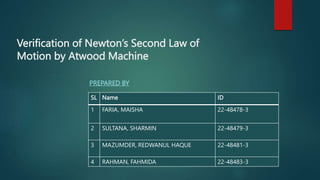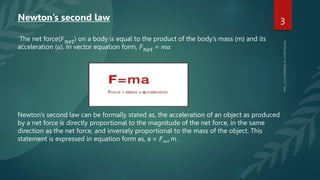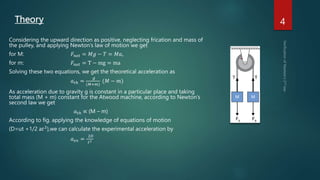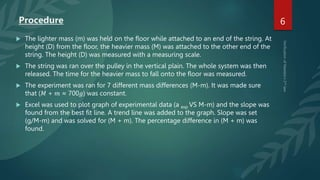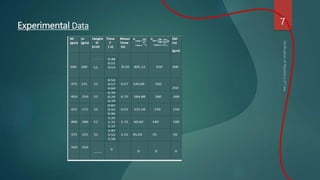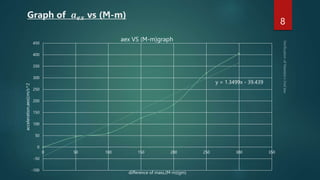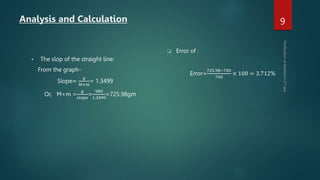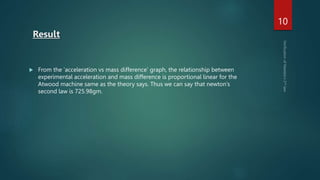1 de 12
Anúncio

### Verification of Newton’s Second Law of Motion. presented by redwanul.pptx

1. Verification of Newton’s Second Law of Motion by Atwood Machine PREPARED BY SL Name ID 1 FARIA, MAISHA 22-48478-3 2 SULTANA, SHARMIN 22-48479-3 3 MAZUMDER, REDWANUL HAQUE 22-48481-3 4 RAHMAN, FAHMIDA 22-48483-3
2. Contents THEORY APPARATUS PROCEDURE EXPERIMENTAL DATA ANALYSIS & CALCULATION RESULT DISCUSSION RESOURCES
3. 3 The net force(𝐹𝑛𝑒𝑡) on a body is equal to the product of the body’s mass (m) and its acceleration (𝑎). In vector equation form, 𝐹𝑛𝑒𝑡 = 𝑚𝑎 Newton's second law can be formally stated as, the acceleration of an object as produced by a net force is directly proportional to the magnitude of the net force, in the same direction as the net force, and inversely proportional to the mass of the object. This statement is expressed in equation form as, a = 𝐹𝑛𝑒𝑡 m . Newton’s second law
4. Theory Considering the upward direction as positive, neglecting frication and mass of the pulley, and applying Newton’s law of motion we get for M: 𝐹𝑛𝑒𝑡 = 𝑀𝑔 − 𝑇 = 𝑀𝑎, for m: 𝐹𝑛𝑒𝑡 = T − mg = ma Solving these two equations, we get the theoretical acceleration as 𝑎𝑡ℎ = 𝑔 (𝑀+𝑚) (𝑀 − 𝑚) As acceleration due to gravity g is constant in a particular place and taking total mass (M + m) constant for the Atwood machine, according to Newton’s second law we get 𝑎𝑡ℎ ∝ (M – m) According to fig. applying the knowledge of equations of motion (D=ut +1/2 a𝑡2 ),we can calculate the experimental acceleration by 𝑎𝑒𝑥 = 2𝐷 𝑡2 4
5. Apparatus  PULLEYS  WEIGHT HANGERS  STRING  METER STICK  STOP WATCH
6. 6 Procedure  The lighter mass (m) was held on the floor while attached to an end of the string. At height (D) from the floor, the heavier mass (M) was attached to the other end of the string. The height (D) was measured with a measuring scale.  The string was ran over the pulley in the vertical plain. The whole system was then released. The time for the heavier mass to fall onto the floor was measured.  The experiment was ran for 7 different mass differences (M-m). It was made sure that (𝑀 + 𝑚 ≈ 700𝑔) was constant.  Excel was used to plot graph of experimental data (a exp VS M-m) and the slope was found from the best fit line. A trend line was added to the graph. Slope was set (g/M-m) and was solved for (M + m). The percentage difference in (M + m) was found.
7. Experimental Data 7
8. Graph of 𝒂𝒆𝒙 vs (M-m) 8 y = 1.3499x - 39.439 -100 -50 0 50 100 150 200 250 300 350 400 450 0 50 100 150 200 250 300 350 acceleration,aex(cm/s^2 difference of mass,(M-m)(gm) aex VS (M-m)graph
9. Analysis and Calculation  The slop of the straight line: From the graph- Slope= 𝑔 𝑀+𝑚 = 1.3499 Or, M+m = 𝑔 𝑠𝑙𝑜𝑝𝑒 = 980 1.3499 =725.98gm  Error of : Error= 725.98−700 700 × 100 = 3.712% 9
10. Result 10  From the ‘acceleration vs mass difference’ graph, the relationship between experimental acceleration and mass difference is proportional linear for the Atwood machine same as the theory says. Thus we can say that newton’s second law is 725.98gm.
11. Discussion  Readings might not have been taken to eye level here measuring height.  Reaction time while taking reading from she-watch might have affected our result.  Take minimum 3 reading of time from a stop-watch and then calculate mean value to minimize errors.  As there are lots of variables, calculation should be done carefully.  The string wasn't moving freely because of some frictional Problem.
12. Thank you!

### Notas do Editor

1. Where, aex = experimental acceleration, D is the distance traveled by the object during time t. If 𝑀 > 𝑚, the acceleration, a, with which the whole system moves is given by, where, ath = theoretical acceleration and (g) is the acceleration due to gravity (9.80 m/s2 ).
Anúncio